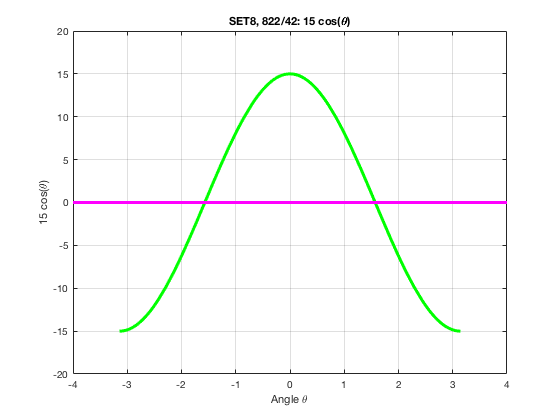# MATH 251: Calculus 3, SET8

## 12: Vectors and the Geometry of Space

### 12.4: The Cross Product

These problems are done with the CAS. See Hand Solutions for details.

#### 1. [821/2]

A cross product of vectors with numerical components is computed. The cross product is orthogonal to the original vectors.
a = sym([4 3 -2]), b = sym([2 -1 1])
a =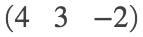b =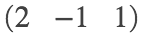c = cross(a,b)
c =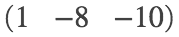a_dot_c = dot(a,c)
a_dot_c = 0
b_dot_c = dot(b,c)
b_dot_c = 0

#### 2. [821/6]

A cross product of vectors with symbolic components is computed.
syms t
a = [t cos(t) sin(t)], b = [1 -sin(t) cos(t)]
a =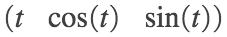b =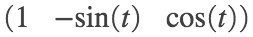c = simplify(cross(a,b))
c =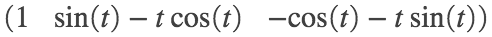#### 3. [821/8]

a = [1 0 -2], b = [0 1 1], c = cross(a,b)
a = 1×3
1 0 -2
b = 1×3
0 1 1
c = 1×3
2 -1 1
figure
quiver3(0,0,0, 1,0,-2); grid on; hold on
quiver3(0,0,0, 0,1,1)
quiver3(0,0,0, 2,-1,1)
axis equal; axis([0 2 -1 1 -2 1])
xlabel('x'); ylabel('y'); zlabel('z')
title('SET8, 821/8')
view(31, 26)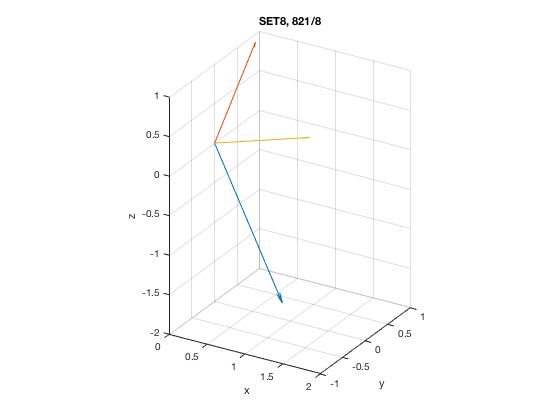#### 4. [821/14]

Recall that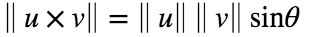. The figure provides the data and we deduce that u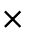v is directed out of the page.
mag_u = 4, mag_v = 5, theta = sym(pi/4)
mag_u = 4
mag_v = 5
theta =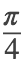mag_u_cross_v = mag_u * mag_v * sin(theta)
mag_u_cross_v =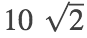#### 5. [821/16]

We can reason from the figure or just assign plausible numerical values to the components of a and b.
So for c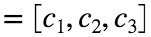, we see that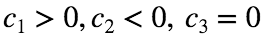.
a = 3*[cosd(60) sind(60), 0]
a = 1×3
1.5000 2.5981 0
b = [0 0 2]
b = 1×3
0 0 2
c = cross(a,b)
c = 1×3
5.1962 -3.0000 0

#### 6. [821/20]

Here we find two unit vectors orthogonal to a given pair of vectors.
u = sym([0 1 -1]), v = sym([1 1 0])
u =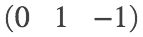v =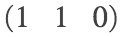c = cross(u,v)
c =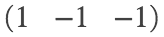w = c/norm(c)
w =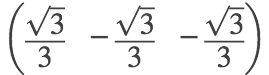neg_w = -w
neg_w =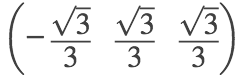#### 7. [821/28]

The area of the parallelogram is the magnitude of the cross product of a pair of edge vectors that span a vertex.
P = sym([1 0 2]), Q = sym([3 3 3]), R = sym([7 5 8]), S = sym([5 2 7])
P =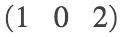Q =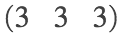R =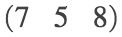S =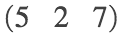PQ = Q-P, PS = S-P
PQ =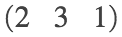PS =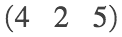c = cross(PQ,PS)
c =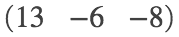V = norm(c)
V =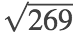V_appx = double(V) % in cm^3
V_appx = 16.4012
%
M = [P; Q; R; S]
M =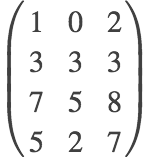x = M(:,1), y = M(:,2), z = M(:,3)
x =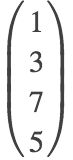y =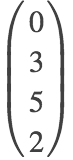z =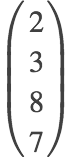x = [x; x(1)]; y = [y; y(1)]; z = [z; z(1)]; % Return to starting vertex!
figure
fill3(x,y,z, 'y'); grid on; hold on
plot3(x,y,z, 'LineWidth', 3)
xlabel('x'); ylabel('y'); zlabel('z')
title('SET8, 821/28')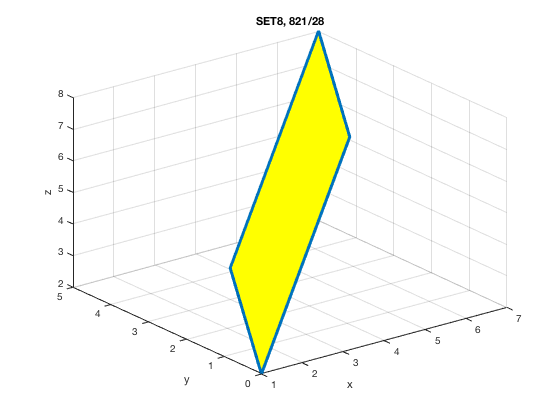#### 8. [822/32]

A normal vector to and the area of a triangle are determined.
P = sym([2 -3 4]), Q = sym([-1, -2 2]), R = sym([3 1 -3])
P =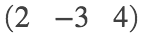Q =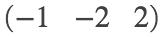R =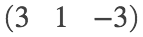PQ = Q-P, PR = R-P
PQ =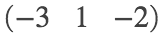PR =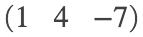n = cross(PQ, PR) % normal (perpendicular) vector to the triangle with vertices P, Q, R
n =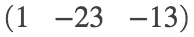A = norm(n)/2 % area of triangle
A =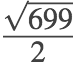A_appx = double(A) % in cm^2
A_appx = 13.2193

#### 9. [822/36]

The volume of a parallelepiped (sheared box) is computed by taking the absolute value of the scalar triple product of a triple of vectors that span a vertex.
P = sym([3 0 1]), Q = sym([-1 2 5]), R = sym([5 1 -1]), S = sym([0 4 2])
P =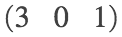Q =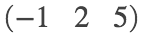R =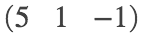S =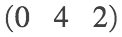PQ = Q-P, PR = R-P, PS = S-P
PQ =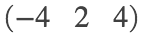PR =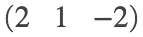PS =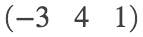c = cross(PR, PS)
c =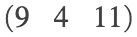V = abs(dot(PQ,c)) % in cm^3
V = 16

#### 10. [822/42]

We see that the magnitude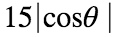of the cross product uv has a maximum of 15 at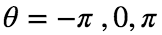. It has a minimum value of 0 at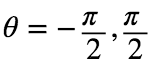. The cross product uv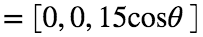points in the direction of k (out of the page) for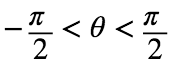and in the direction of -k (into the page) for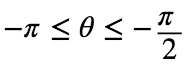or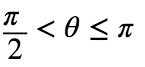.
syms theta
u = 3*[cos(theta), sin(theta) 0], v = sym([0 5 0])
u =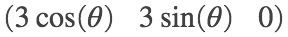v =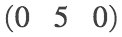c = cross(u,v)
c =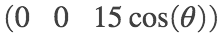mag_c = simplify(norm(c))
mag_c =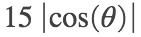figure
fplot(mag_c, [-pi pi], 'LineWidth', 3); grid on; hold on
axis([-4 4 -5 20])
xlabel('Angle \theta'); ylabel('15 | cos(\theta) |')
title('SET8, 822/42: || u x v ||')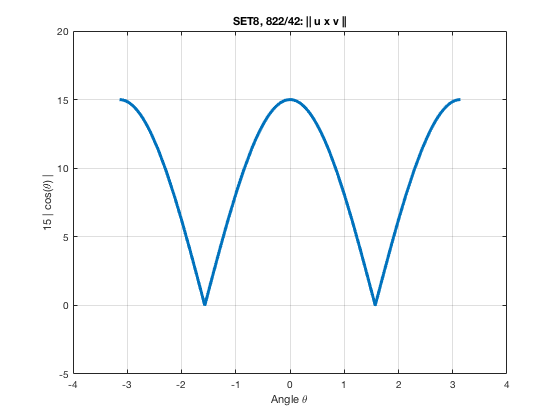%
figure
fplot(15*cos(theta), [-pi pi], 'g', 'LineWidth', 3); grid on; hold on
plot([-4 4], [0 0], 'm', 'LineWidth', 3)
axis([-4 4 -20 20])
xlabel('Angle \theta'); ylabel('15 cos(\theta)')
title('SET8, 822/42: 15 cos(\theta)')